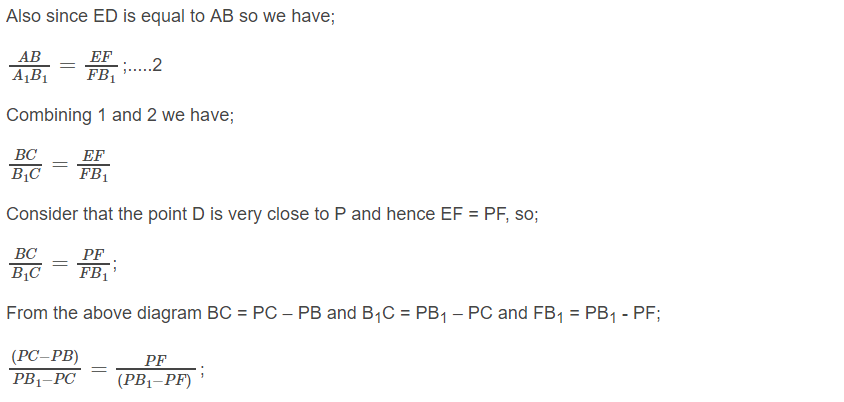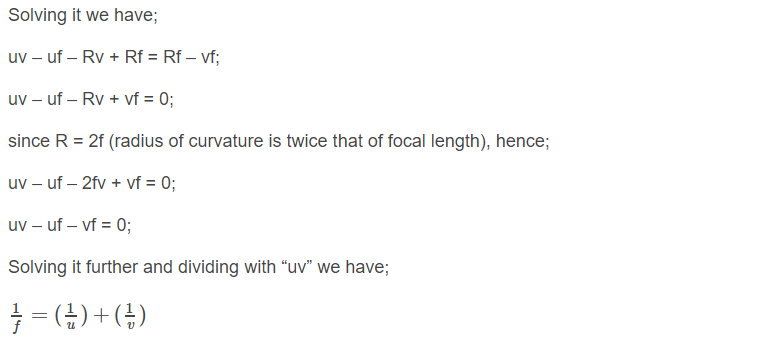# Derivation Of Mirror FormulaThe derivation of the mirror formula is one of the most common questions asked in various board examinations as well as competitive examinations. A mirror formula can be defined as the formula which gives the relationship between the distance of object ‘u’, the distance of image ‘v’, and the focal length of the mirror ‘f’. The mirror formula is applicable for both, plane mirrors and spherical mirrors (convex and concave mirrors). The mirror formula derivation is provided here so that students can understand the concept of the topic in a better way. The mirror formula is written as:## Assumptions for Derivation Of Mirror Formula

The following assumptions are taken in order to derive the mirror formula.

• The distances are being measured from the pole of the mirror.
• According to the convention, the negative sign indicates the distance measured in the direction opposite to the incident ray while the positive sign indicates the distance measured in the direction of the incident ray.
• The distance below the axis is negative whereas the distance above is positive.

## Mirror Formula Derivation

The derivation of mirror formula is given below. The diagram given below will help learners to understand the mirror formula derivation more effectively.From the figure given above, it is obvious that the object AB is placed at a distance of U from P which is the pole of the mirror. From the diagram we can also say that the image A1B1 is formed at V from the mirror.

Now from the above diagram, it is clear that according to the law of vertically opposite angles the opposite angles are equal. So we can write:This was the derivation of mirror formula. Students must understand each step of the mirror formula derivation to get a piece of in-depth knowledge about the topic. Stay tuned with BYJU’S and learn various other derivation of physics formulas.

Test your knowledge on Mirror formula derivation

1. it good
thanks

1. Subhashree

Nice explanation ..

2. Mahendar kumar

This website really helped me a lot in my exams and I also learnt such new things which our teacher had never taught us!